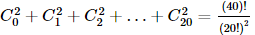Courses

# In the following question, a Statement of Assertion (A) is given followed by a corresponding Reason (R) just below it. Read the Statements carefully and mark the correct answer-Assertion(A):If Cr is the coefficient of xr in the expansion of (1 + x)20Reason(R) : Cr = Cn − r for any positive integer na)Assertion is true but Reason is false.b)Both Assertion and Reason are true and Reason is not the correct explanation of Assertion.c)Both Assertion and Reason are true and Reason is the correct explanation of Assertion.d)Assertion is false but Reason is true.Correct answer is option 'A'. Can you explain this answer? Related Test: JEE(MAIN) Mathematics Mock Test - 1

## JEE Question

By Robins Kumar · May 22, 2020 ·JEE
Assertion is correct but the reason is not becoz in reason this concept is not use ..the use concet is that (1+×)²⁰=(×+1)²⁰..so reson is not correct

Harsh Singhal answered Mar 02, 2020
Reason is false bcz it must be req.to say that n-r>0
& r less than or equal to n

Khushi Sanghvi answered Mar 20, 2020
How can be the assertion is corrct? pls anyone send the soln